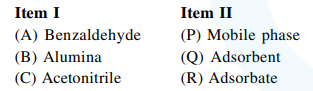Deepak Scored 45->99%ile with Bounce Back Crack Course. You can do it too!

# The correct match between Item I and Item II is:

Question:

The correct match between Item I and Item II is:1. $(\mathrm{A}) \rightarrow(\mathrm{Q}) ;(\mathrm{B}) \rightarrow(\mathrm{R}) ;(\mathrm{C}) \rightarrow(\mathrm{P})$

2. $(\mathrm{A}) \rightarrow(\mathrm{P}) ;(\mathrm{B}) \rightarrow(\mathrm{R}) ;(\mathrm{C}) \rightarrow(\mathrm{Q})$

3. $(\mathrm{A}) \rightarrow(\mathrm{Q}) ;(\mathrm{B}) \rightarrow(\mathrm{P}) ;(\mathrm{C}) \rightarrow(\mathrm{R})$

4. $(\mathrm{A}) \rightarrow(\mathrm{R}) ;(\mathrm{B}) \rightarrow(\mathrm{Q}) ;(\mathrm{C}) \rightarrow(\mathrm{P})$

Correct Option: , 4

Solution:

$(\mathrm{A}) \rightarrow(\mathrm{R}) ;(\mathrm{B}) \rightarrow(\mathrm{Q}) ;(\mathrm{C}) \rightarrow(\mathrm{P})$